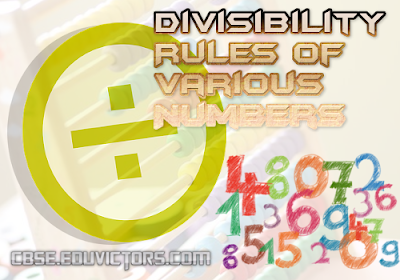## Divisibility Rules of Various Numbers

(2-25)

Divisibility by 2:
A number is divisible by 2 if its unit’s digit is even or 0.

Divisibility by 3:
A number is divisible by 3 if the sum of its digits are divisible by 3.

Divisibility by 4:
A number is divisible by 4 if the last 2 digits are divisible by 4, or if the last two digits are 0’s.

Divisibility by 5:
A number is divisible by 5 if its unit’s digit is 5 or 0.

Divisibility by 6:
A number is divisible by 6 if it is simultaneously divisible by 2 and 3.

Divisibility by 7:
A number is divisible by 7 if unit’s place digit is multiplied by 2 and subtracted from the remaining digits and the number obtained is divisible by 7.

Divisibility by 8:
A number is divisible by 8 if the last 3 digits of the number are divisible by 8, or if the last three digits of a number are zeros.

Divisibility by 9:
A number is divisible by 9 if the sum of its digits is divisible by 9.

Divisibility by 10:
A number is divisible by 10 if its unit’s digit is 0.

Divisibility by 11:
A number is divisible by 11 if the sum of digits at odd and even places are equal or differ by a number divisible by 11.

Divisibility by 12:
A number is divisible by 12 if the number is divisible by both 4 and 3.

Divisibility by 13:
A number is divisible by 13 if its unit’s place digit is multiplied by 4 and added to the remaining digits and the number obtained is divisible by 13.

Divisibility by 14 :
A number is divisible by 14 if the number is divisible by both 2 and 7.

Divisibility by 15:
A number is divisible by 15 if the number is divisible by both 3 and 5.

Divisibility by 16:
A number is divisible by 16 if its last 4 digits is divisible by 16 or if the last four digits are zeros.

Divisibility by 17:
A number is divisible by 17 if its unit’s place digit is multiplied by 5 and subtracted from the remaining digits and the number obtained is divisible by 17.

Divisibility by 18:
A number is divisible by 18 if the number is divisible by both 2 and 9.

Divisibility by 19:
A number is divisible by 19 if its unit’s place digit is multiplied by 2 and added to the remaining digits and the number obtained is divisible by 19.

Rule for Divisibility by 25:
A number is divisible by 25 if it ends with 00, 25, 50, or 75.

Q1: Is 112 divisible by 7?

Solution:
Step I : Separate the last digit & multiply with two and then subtract
112 : 11 - 2 × 2 = 7
∵ 7 is divisible by 7. 112 is divisible by 7.

Q2: Is 2961 divisible by 7?

Solution:
Step I : 296 - 1 × 2 = 294
Step II :  29 - 4 × 2 = 21
∵ 21 is divisible by 7. 2961 is divisible by 7.

Q3: Is 143 divisible by 13 ?